This is “Review Exercises and Sample Exam”, section 3.9 from the book Beginning Algebra (v. 1.0). For details on it (including licensing), click here.

Has this book helped you? Consider passing it on:
Creative Commons supports free culture from music to education. Their licenses helped make this book available to you.
DonorsChoose.org helps people like you help teachers fund their classroom projects, from art supplies to books to calculators.

## 3.9 Review Exercises and Sample Exam

### Review Exercises

Rectangular Coordinate System

Graph the given set of ordered pairs.

1. ${(−3, 4), (−4, 0), (0, 3), (2, 4)}$

2. ${(−5, 5), (−3, −1), (0, 0), (3, 2)}$

3. Graph the points (−3, 5), (−3, −3), and (3, −3) on a rectangular coordinate plane. Connect the points and calculate the area of the shape.

4. Graph the points (−4, 1), (0, 1), (0, −2), and (−4, −2) on a rectangular coordinate plane. Connect the points and calculate the area of the shape.

5. Graph the points (1, 0), (4, 0), (1, −5), and (4, −5) on a rectangular coordinate plane. Connect the points and calculate the perimeter of the shape.

6. Graph the points (−5, 2), (−5, −3), (1, 2), and (1, −3) on a rectangular coordinate plane. Connect the points and calculate the perimeter of the shape.

Calculate the distance between the given two points.

7. (−1, −2) and (5, 6)

8. (2, −5) and (−2, −2)

9. (−9, −3) and (−8, 4)

10. (−1, 3) and (1, −3)

Calculate the midpoint between the given points.

11. (−1, 3) and (5, −7)

12. (6, −3) and (−8, −11)

13. (7, −2) and (−6, −1)

14. (−6, 0) and (0, 0)

15. Show algebraically that the points (−1, −1), (1, −3), and (2, 0) form an isosceles triangle.

16. Show algebraically that the points (2, −1), (6, 1), and (5, 3) form a right triangle.

Graph by Plotting Points

Determine whether the given point is a solution.

17. $−5x+2y=7$; $(1, −1)$

18. $6x−5y=4$; $(−1, −2)$

19. $y=34x+1$; $(−23, 12)$

20. $y=−35x−2$; $(10, −8)$

Find at least five ordered pair solutions and graph.

21. $y=−x+2$

22. $y=2x−3$

23. $y=12x−2$

24. $y=−23x$

25. $y=3$

26. $x=−3$

27. $x−5y=15$

28. $2x−3y=12$

Graph Using Intercepts

Given the graph, find the x- and y- intercepts.

29.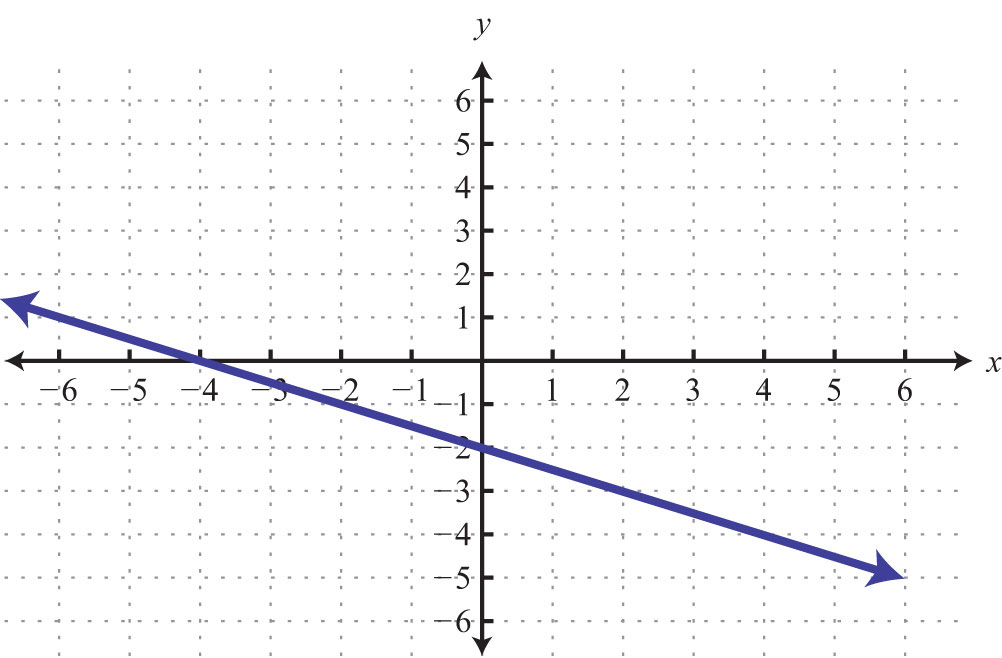30.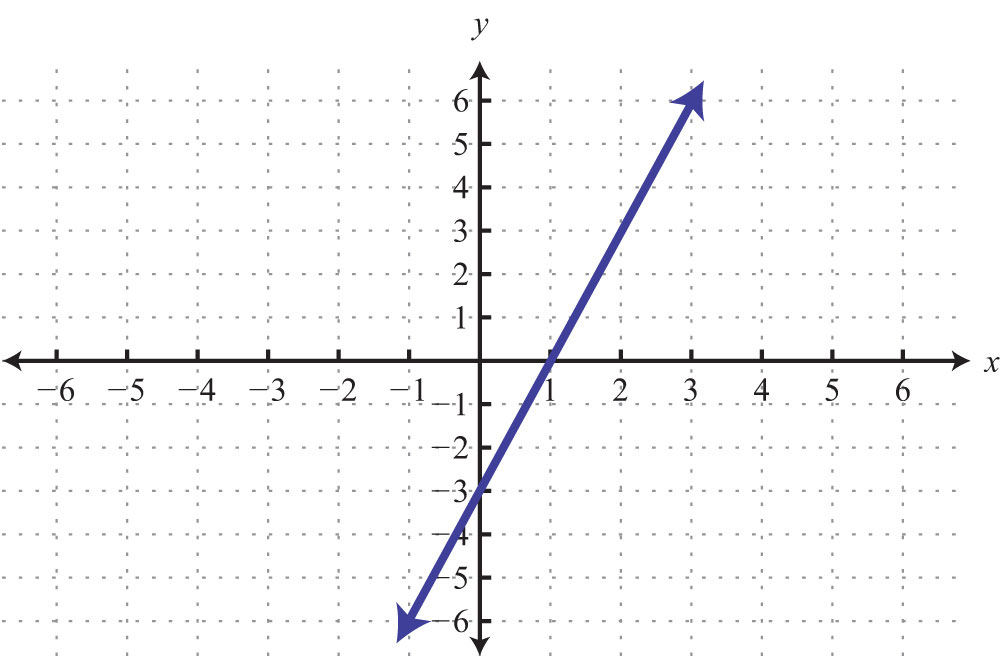31.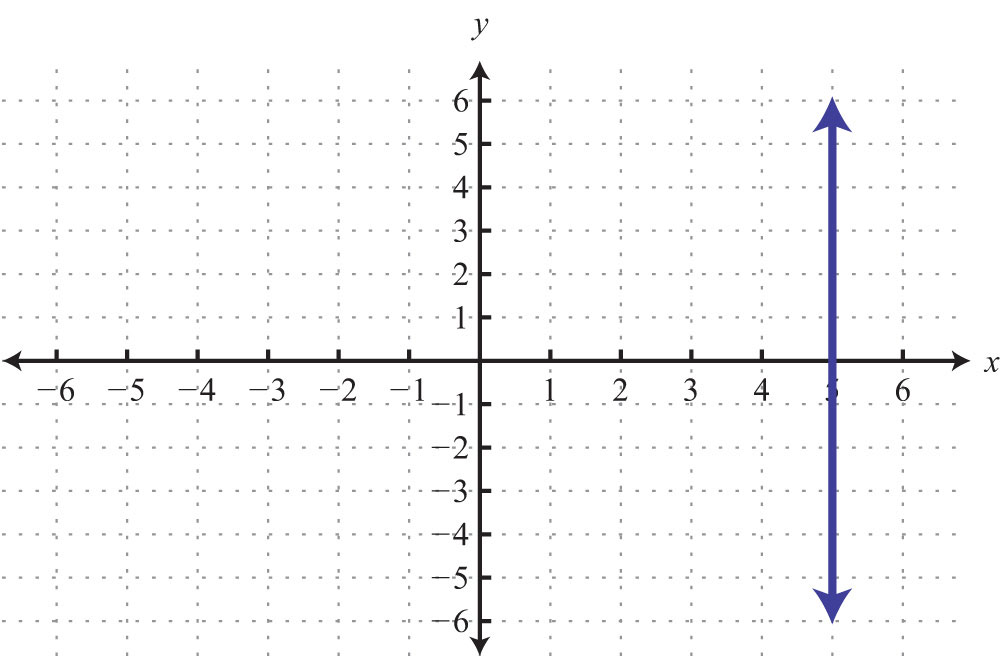32.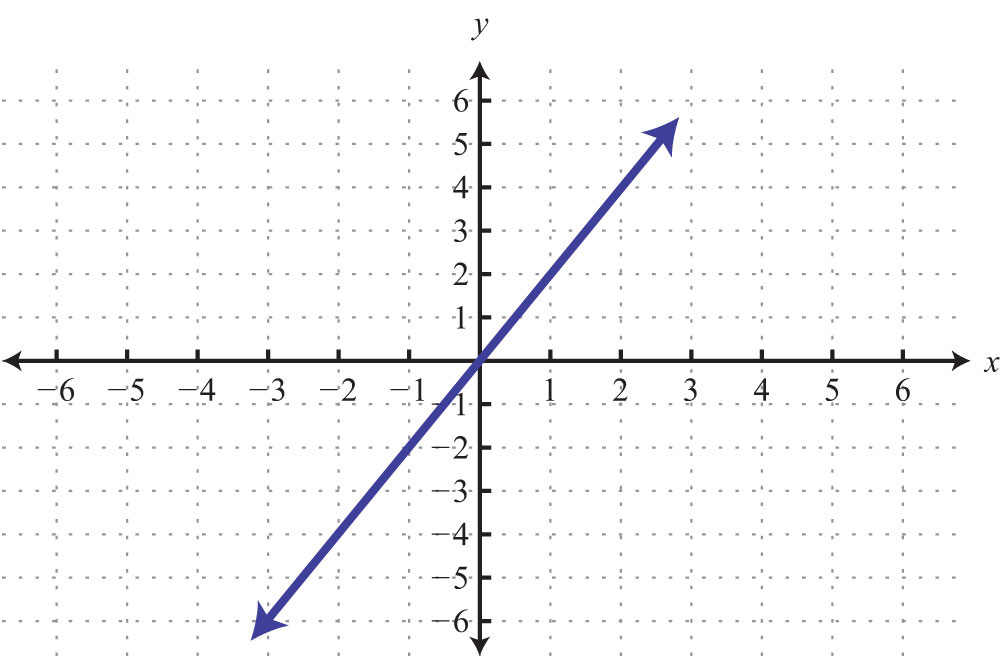Find the intercepts and graph them.

33. $3x−4y=12$

34. $2x−y=−4$

35. $12x−13y=1$

36. $−12x+23y=2$

37. $y=−53x+5$

38. $y=−3x+4$

Graph Using the y-Intercept and Slope

Given the graph, determine the slope and y-intercept.

39.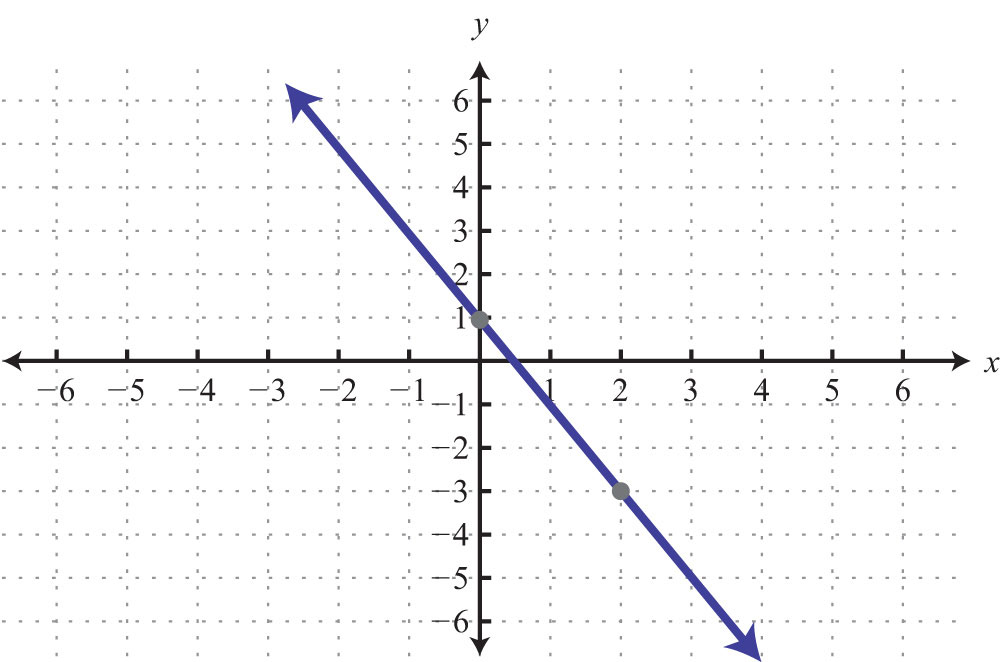40.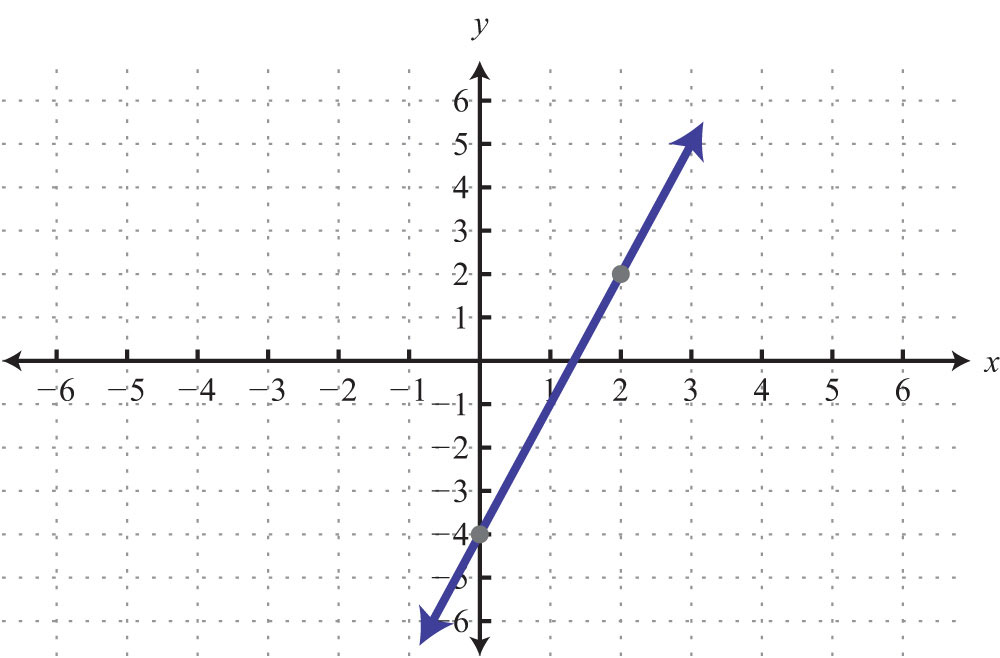Determine the slope, given two points.

41. (−3, 8) and (5, −6)

42. (0, −5) and (−6, 3)

43. (1/2, −2/3) and (1/4, −1/3)

44. (5, −3/4) and (2, −3/4)

Express in slope-intercept form and identify the slope and y-intercept.

45. $12x−4y=8$

46. $3x−6y=24$

47. $−13x+34y=1$

48. $−5x+3y=0$

Graph using the slope and y-intercept.

49. $y=−x+3$

50. $y=4x−1$

51. $y=−2x$

52. $y=−52x+3$

53. $2x−3y=9$

54. $2x+32y=3$

55. $y=0$

56. $x−4y=0$

Finding Linear Equations

Given the graph, determine the equation of the line.

57.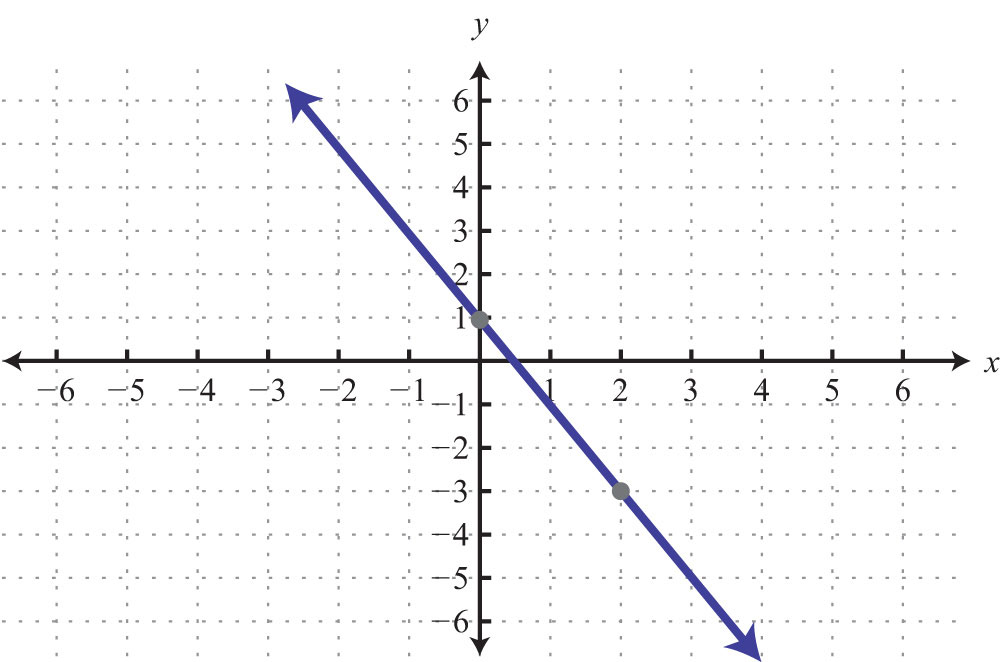58.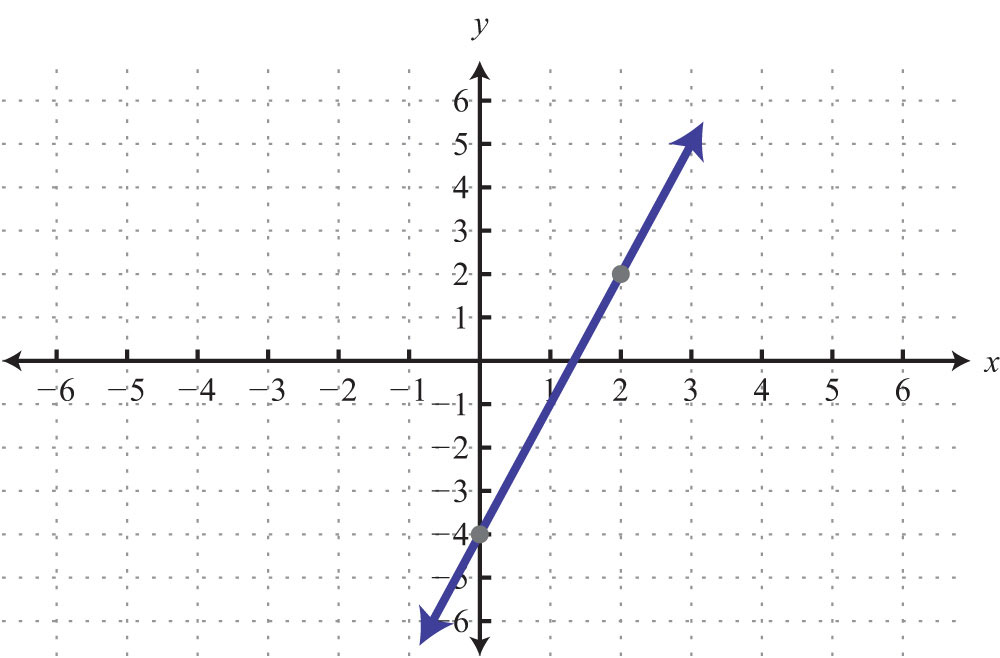59.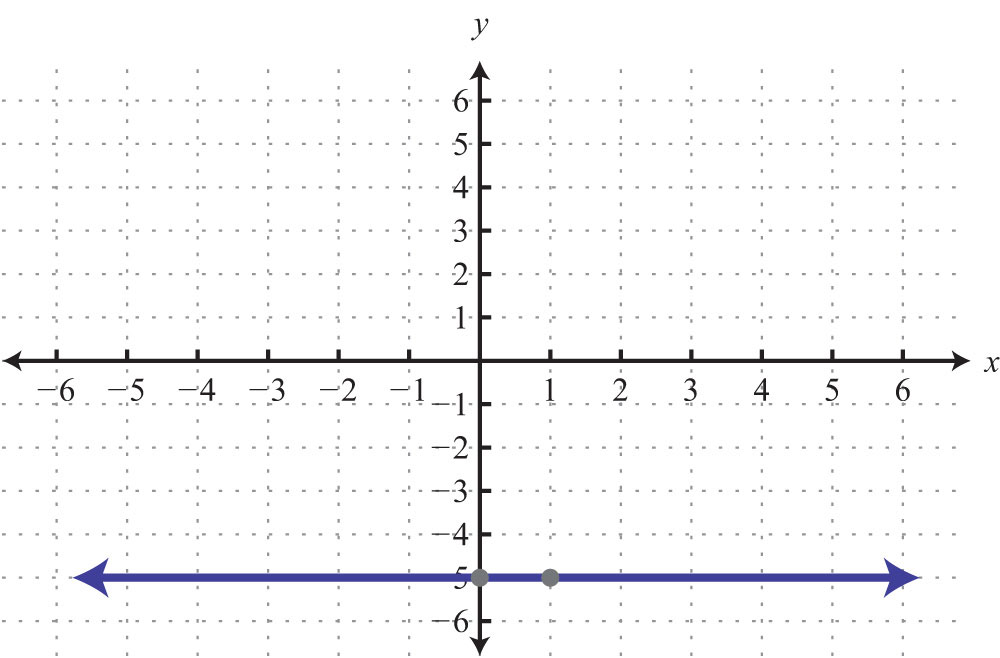60.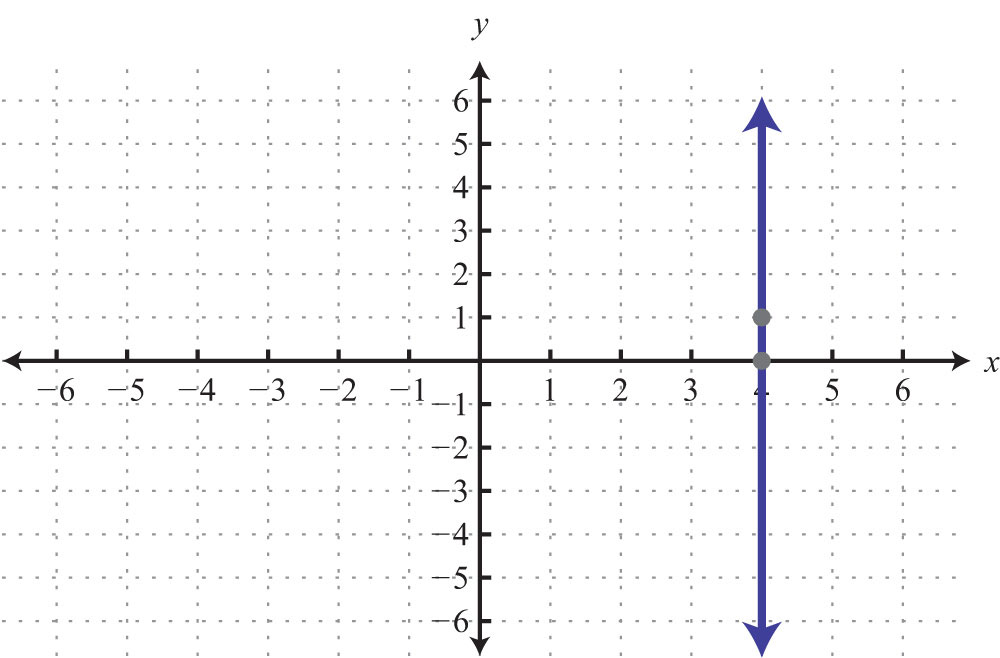Find the equation of a line, given the slope and a point on the line.

61. m = 1/2; (−4, 8)

62. m = −1/5; (−5, −9)

63. m = 2/3; (1, −2)

64. m = −3/4; (2, −3)

Find the equation of the line given two points on the line.

65. (−5, −5) and (10, 7)

66. (−6, 12) and (3, −3)

67. (2, −1) and (−2, 2)

68. (5/2, −2) and (−5, 5/2)

69. (7, −6) and (3, −6)

70. (10, 1) and (10, −3)

Parallel and Perpendicular Lines

Determine if the lines are parallel, perpendicular, or neither.

71. ${−3x+7y=146x−14y=42$

72. ${2x+3y=182x−3y=36$

73. ${x+4y=28x−2y=−1$

74. ${y=2x=2$

Find the equation of the line in slope-intercept form.

75. Parallel to $5x−y=15$ and passing through (−10, −1).

76. Parallel to $x−3y=1$ and passing through (2, −2).

77. Perpendicular to $8x−6y=4$ and passing through (8, −1).

78. Perpendicular to $7x+y=14$ and passing through (5, 1).

79. Parallel to $y=1$ and passing through (4, −1).

80. Perpendicular to $y=1$ and passing through (4, −1).

Introduction to Functions

Determine the domain and range and state whether it is a function or not.

81. {(−10, −1), (−5, 2), (5, 2)}

82. {(−12, 4), (−1, −3), (−1, −2)}

83.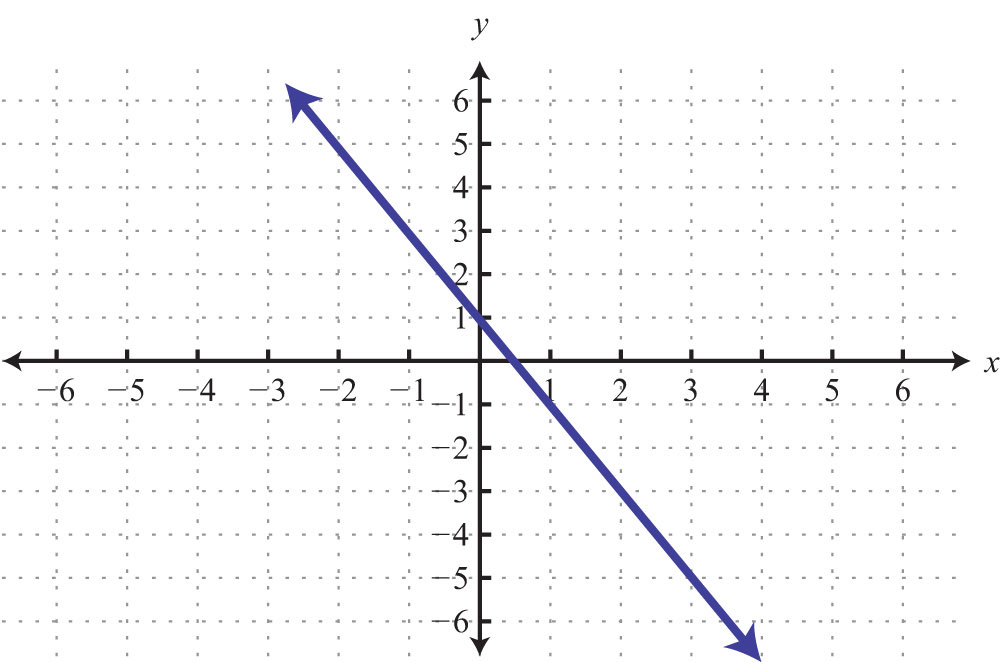84.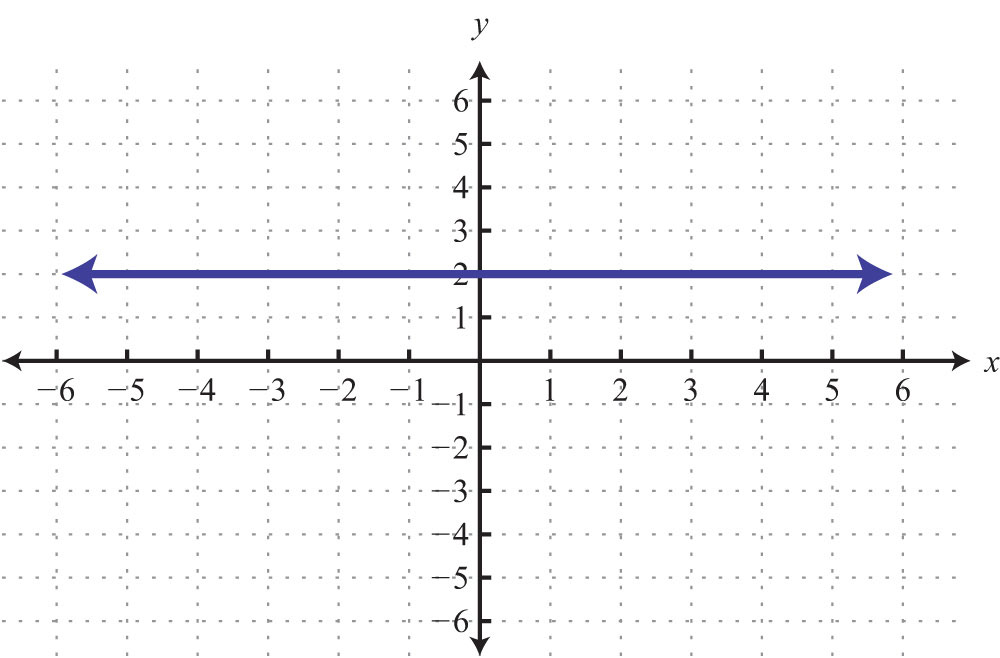85.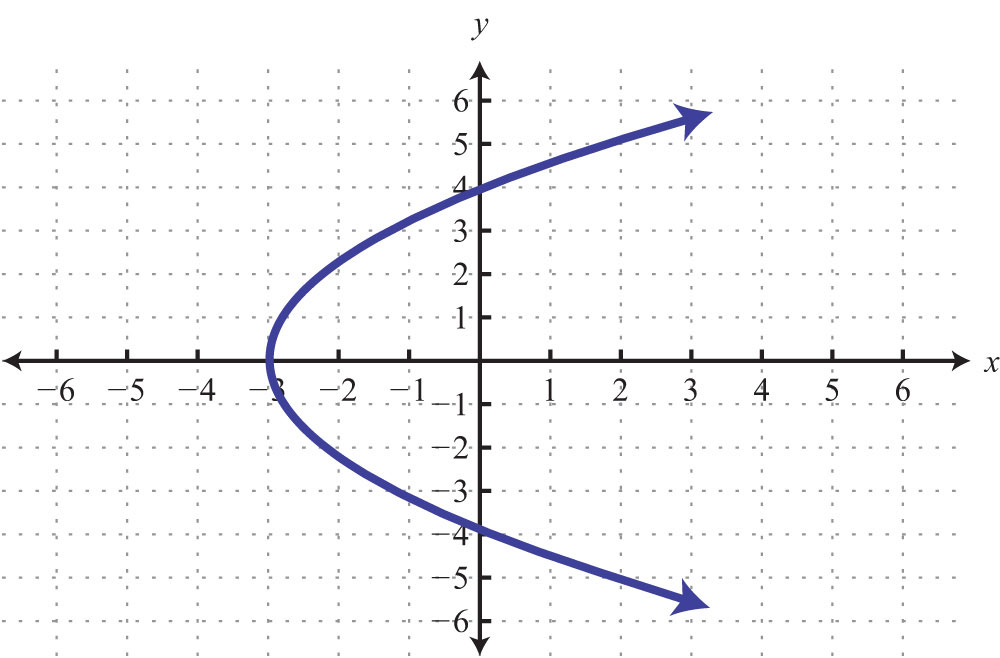86.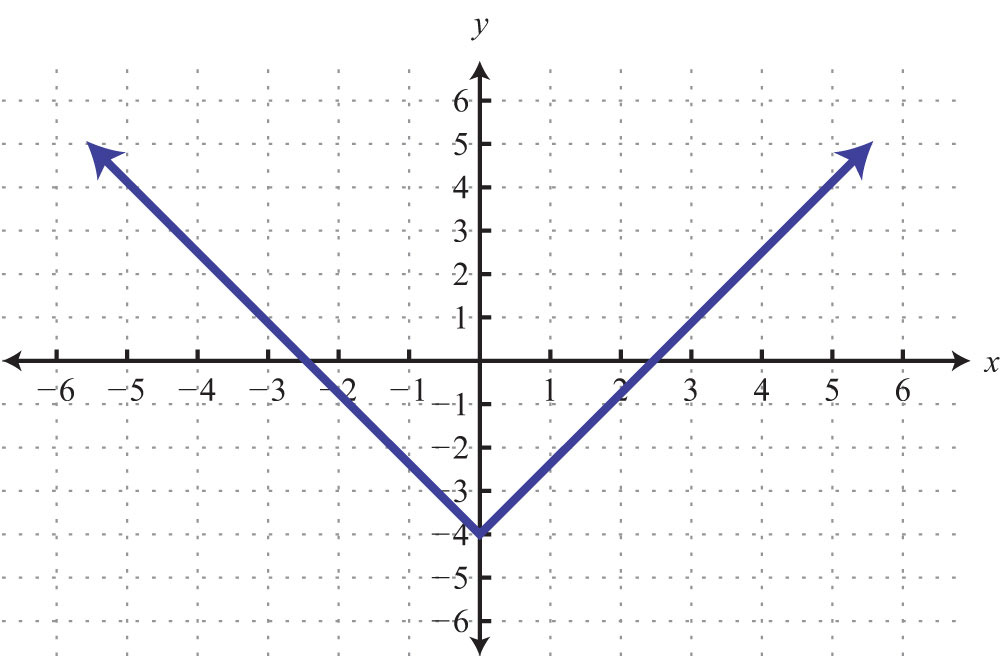Given the following,

87. $f(x)=9x−4$, find $f(−1)$.

88. $f(x)=−5x+1$, find $f(−3)$.

89. $g(x)=12x−13$, find $g(−13)$.

90. $g(x)=−34x+13$, find $g(23)$.

91. $f(x)=9x−4$, find x when $f(x)=0$.

92. $f(x)=−5x+1$, find x when $f(x)=2$.

93. $g(x)=12x−13$, find x when $g(x)=1$.

94. $g(x)=−34x+13$, find x when $g(x)=−1$.

Given the graph of a function $f(x)$, determine the following.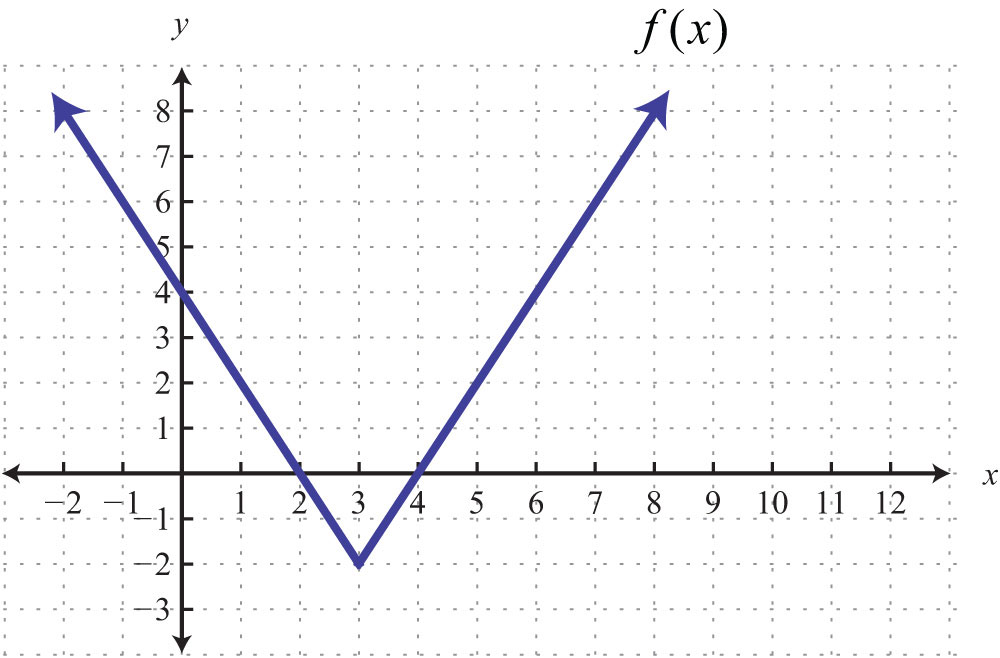95. $f(3)$

96. x when $f(x)=4$

Linear Inequalities (Two Variables)

Is the ordered pair a solution to the given inequality?

97. $6x−2y≤1$; (−3, −7)

98. $−3x+y>2$; (0, 2)

99. $6x−10y<−1$; (5, −3)

100. $x−13y>0$; (1, 4)

101. $y>0$; (−3, −1)

102. $x≤−5$; (−6, 4)

Graph the solution set.

103. $y≥−2x+1$

104. $y<3x−4$

105. $−x+y≤3$

106. $52x+12y≤2$

107. $3x−5y>0$

108. $y>0$

### Sample Exam

1. Graph the points (−4, −2), (−4, 1), and (0, −2) on a rectangular coordinate plane. Connect the points and calculate the area of the shape.

2. Is (−2, 4) a solution to $3x−4y=−10$? Justify your answer.

Given the set of x-values {−2, −1, 0, 1, 2}, find the corresponding y-values and graph the following.

3. $y=x−1$

4. $y=−x+1$

5. On the same set of axes, graph $y=4$ and $x=−3$. Give the point where they intersect.

Find the x- and y-intercepts and use those points to graph the following.

6. $2x−y=8$

7. $12x+5y=15$

8. Calculate the slope of the line passing through (−4, −5) and (−3, 1).

Determine the slope and y-intercept. Use them to graph the following.

9. $y=−32x+6$

10. $5x−2y=6$

11. Given $m=−3$, determine $m⊥$.

12. Are the given lines parallel, perpendicular, or neither? ${−2x+3y=−124x−6y=30$

13. Determine the slope of the given lines.

a. $y=−2$

b. $x=13$

c. Are these lines parallel, perpendicular, or neither?

15. Determine the equation of the line with slope $m=−34$ passing through (8, 1).

16. Find the equation to the line passing through (−2, 3) and (4, 1).

17. Find the equation of the line parallel to $5x−y=6$ passing through (−1, −2).

18. Find the equation of the line perpendicular to $−x+2y=4$ passing through (1/2, 5).

Given a linear function $f(x)=−45x+2$, determine the following.

19. $f(10)$

20. x when $f(x)=0$

21. Graph the solution set: $3x−4y>4$.

22. Graph the solution set: $y−2x≥0$.

23. A rental car company charges $32.00 plus$0.52 per mile driven. Write an equation that gives the cost of renting the car in terms of the number of miles driven. Use the formula to determine the cost of renting the car and driving it 46 miles.

24. A car was purchased new for $12,000 and was sold 5 years later for$7,000. Write a linear equation that gives the value of the car in terms of its age in years.

25. The area of a rectangle is 72 square meters. If the width measures 4 meters, then determine the length of the rectangle.

1: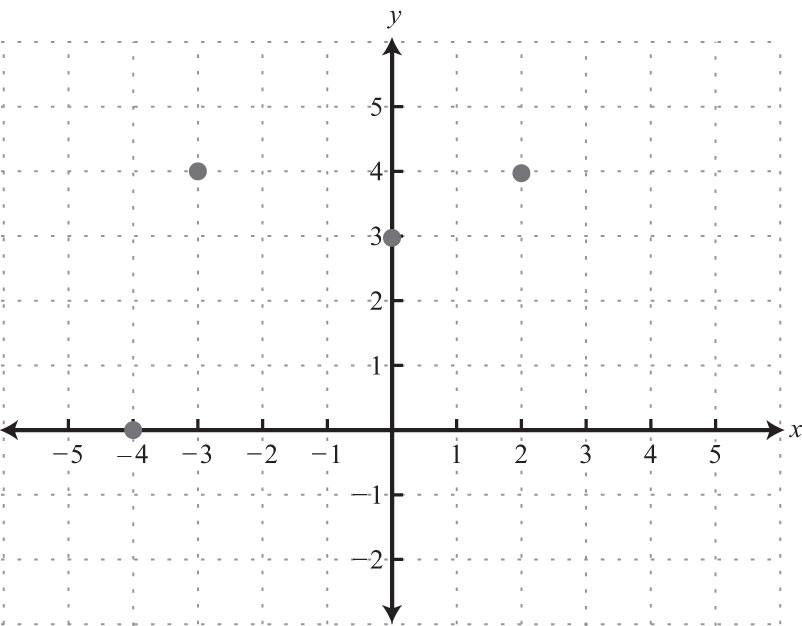3: Area: 24 square units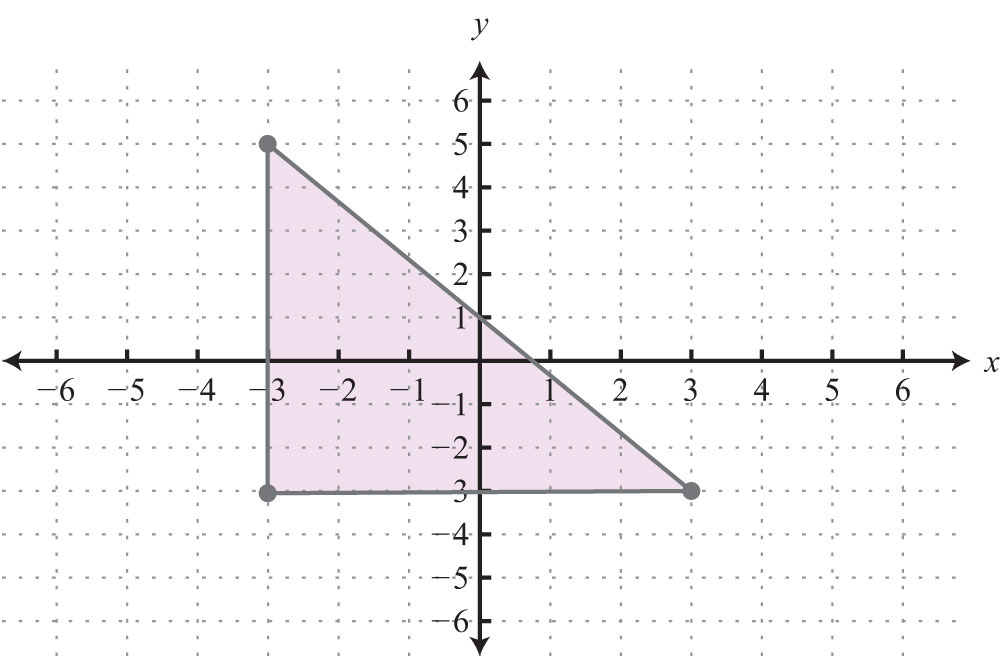5: Perimeter: 16 units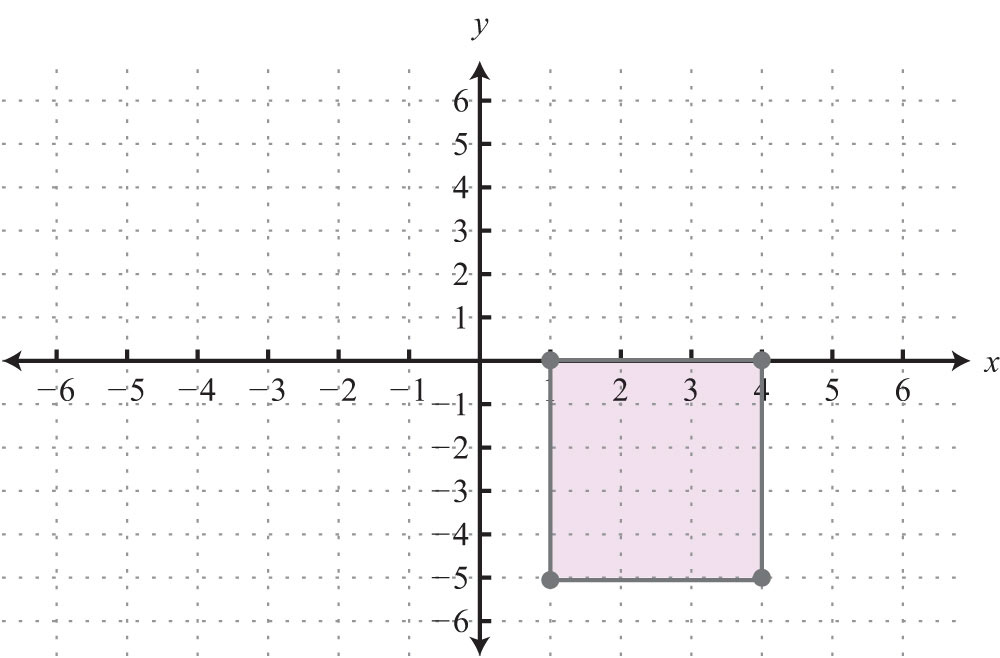7: 10 units

9: $52$ units

11: (2, −2)

13: (1/2, −3/2)

17: No

19: Yes

21: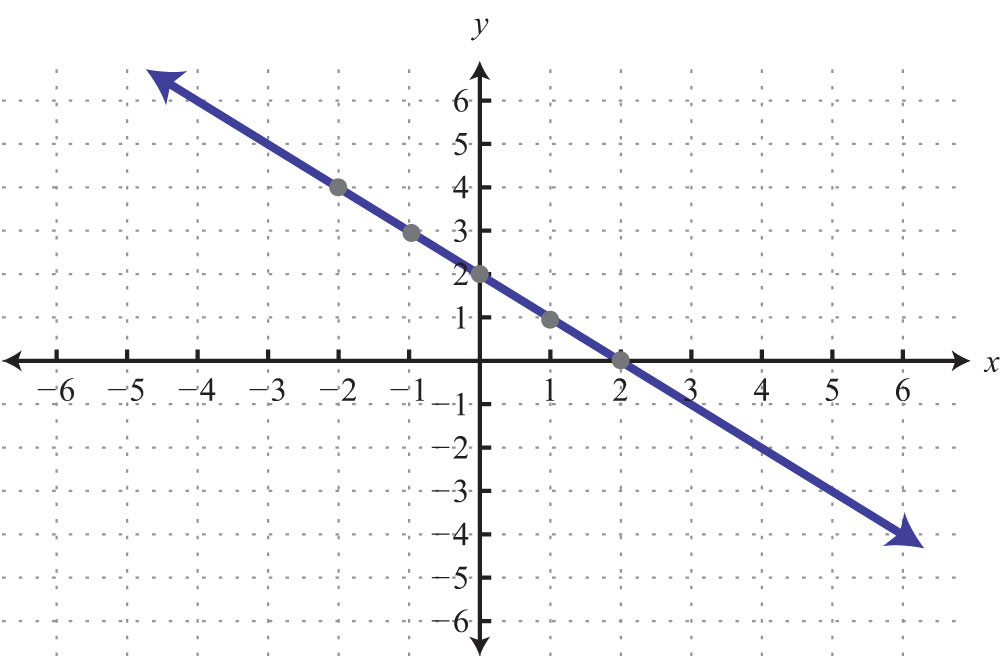23: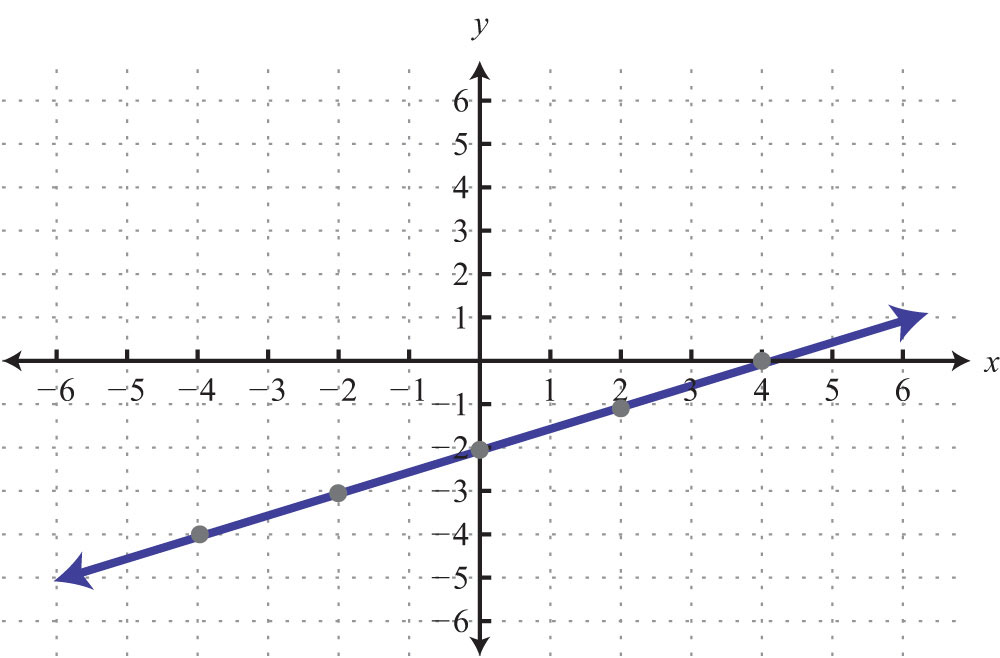25: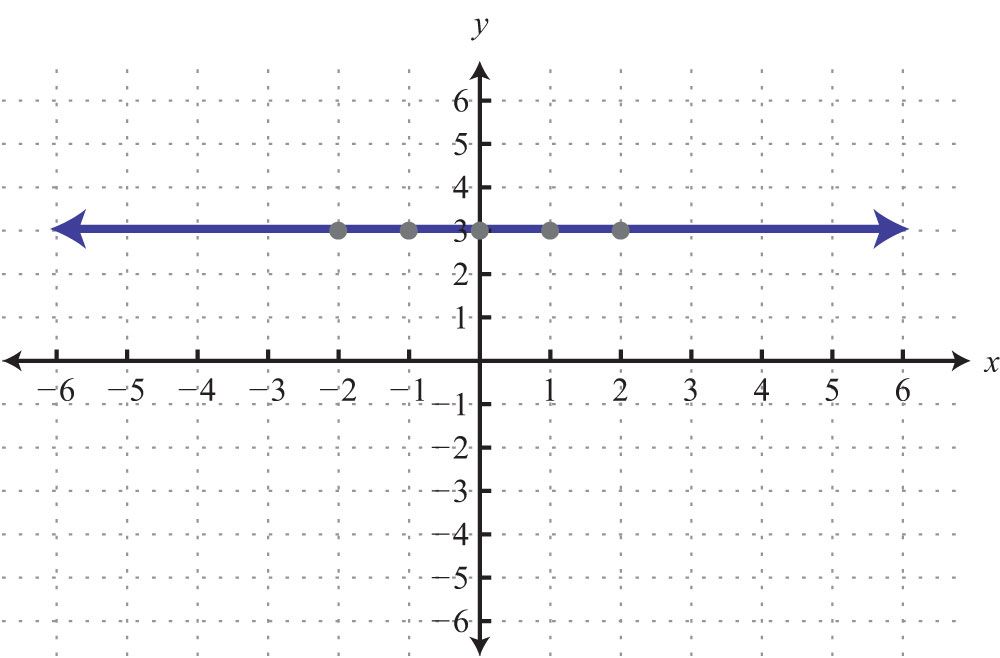27: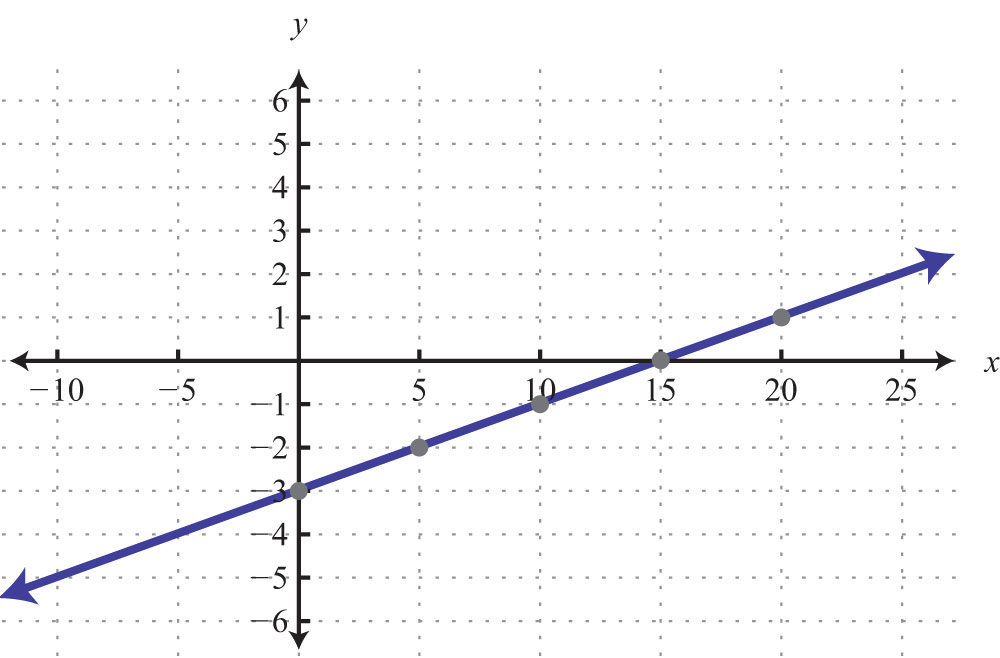29: y-intercept: (0, −2); x-intercept: (−4, 0)

31: y-intercept: none; x-intercept: (5, 0)

33: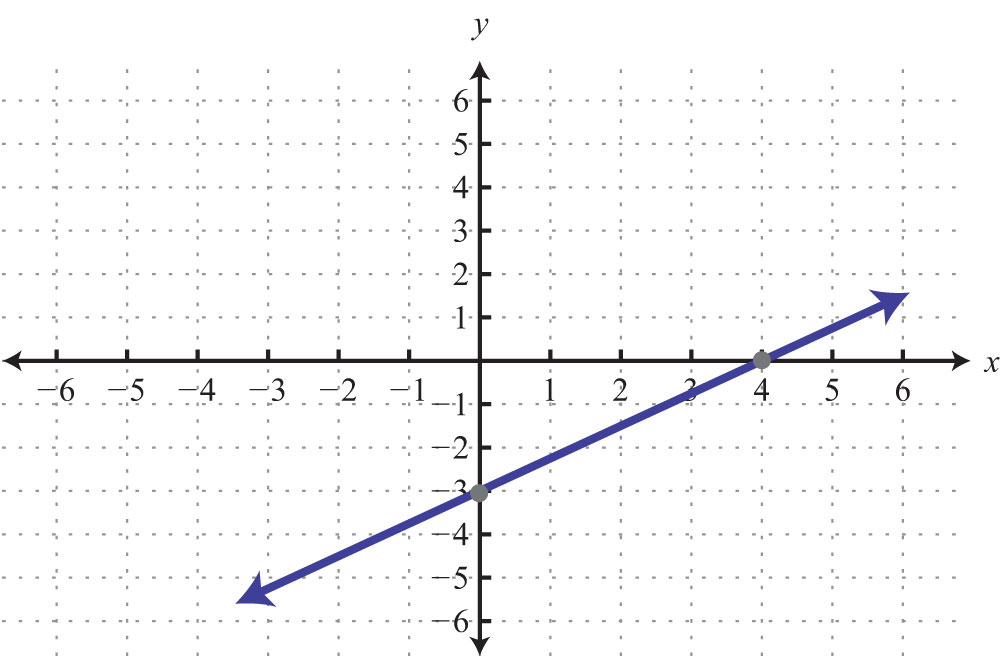35: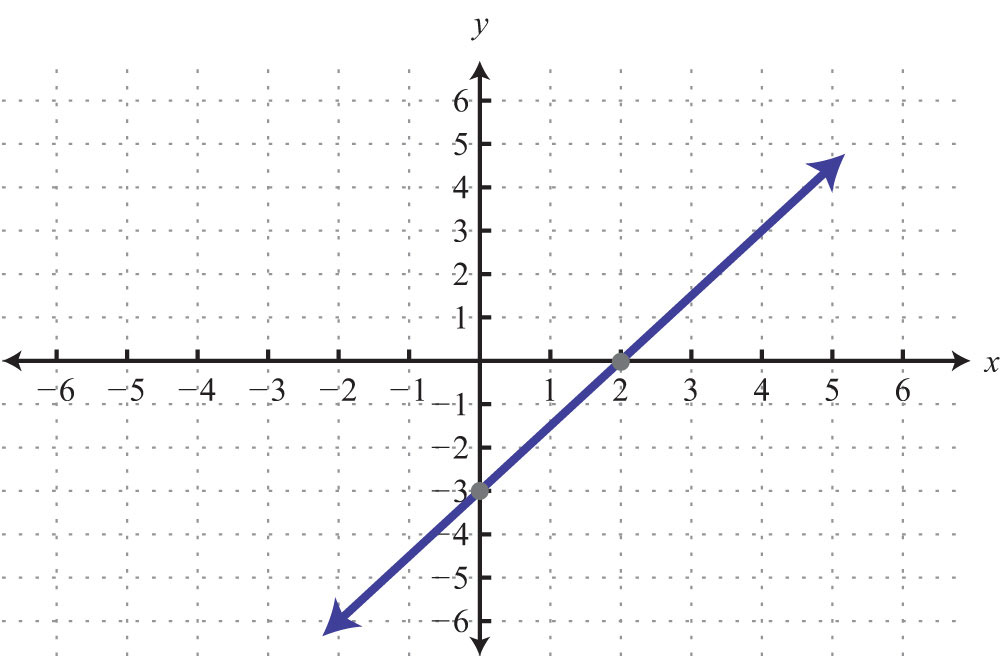37: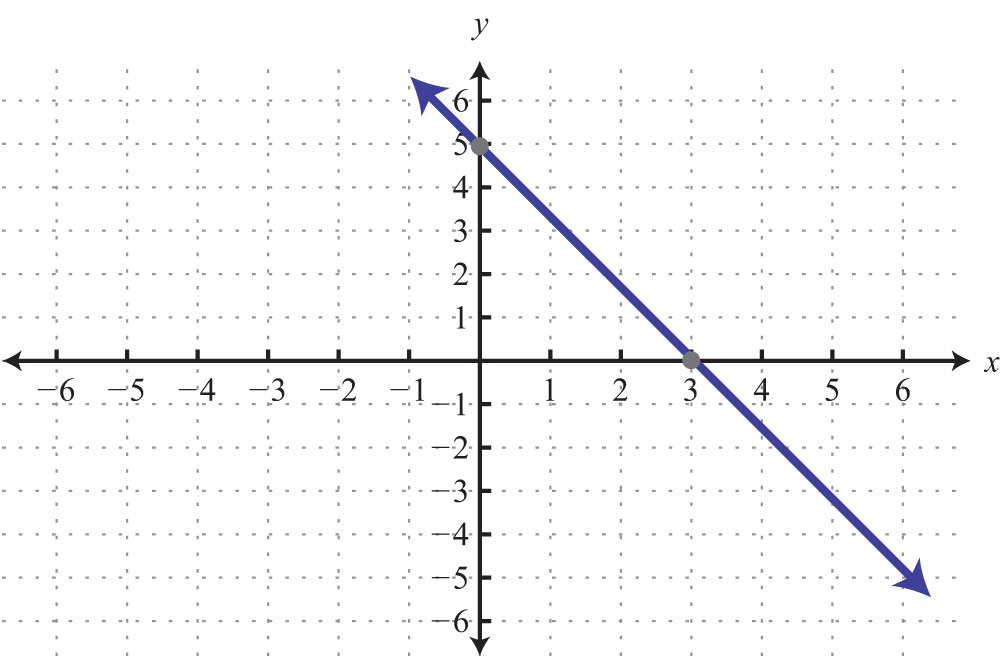39: y-intercept: (0, 1); slope: −2

41: −7/4

43: −4/3

45: $y=3x−2$; slope: 3; y-intercept (0, −2)

47: $y=49x+43$; slope: 4/9; y-intercept (0, 4/3)

49: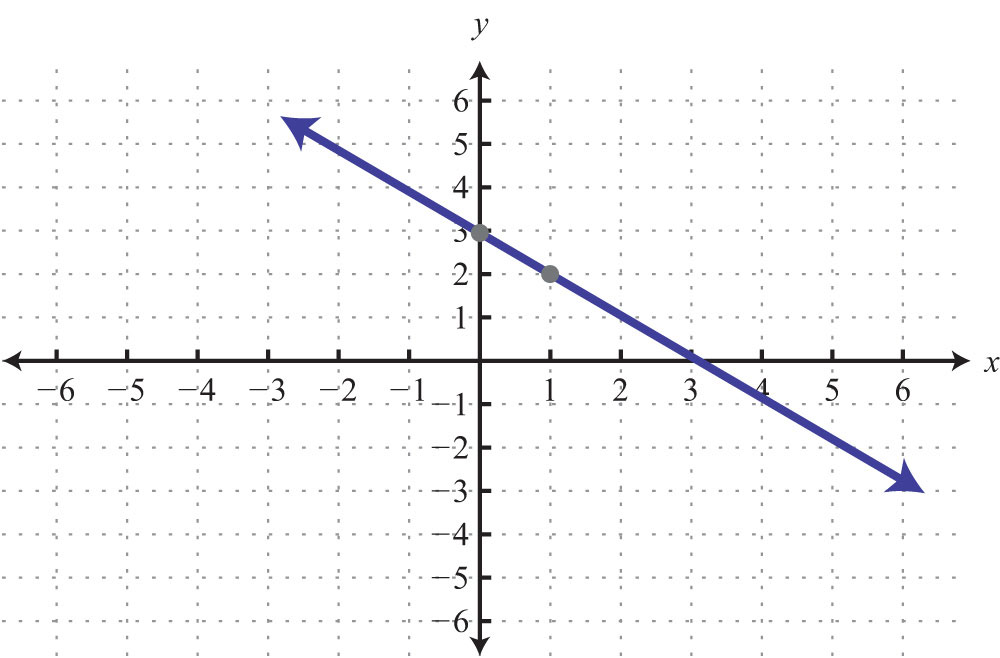51: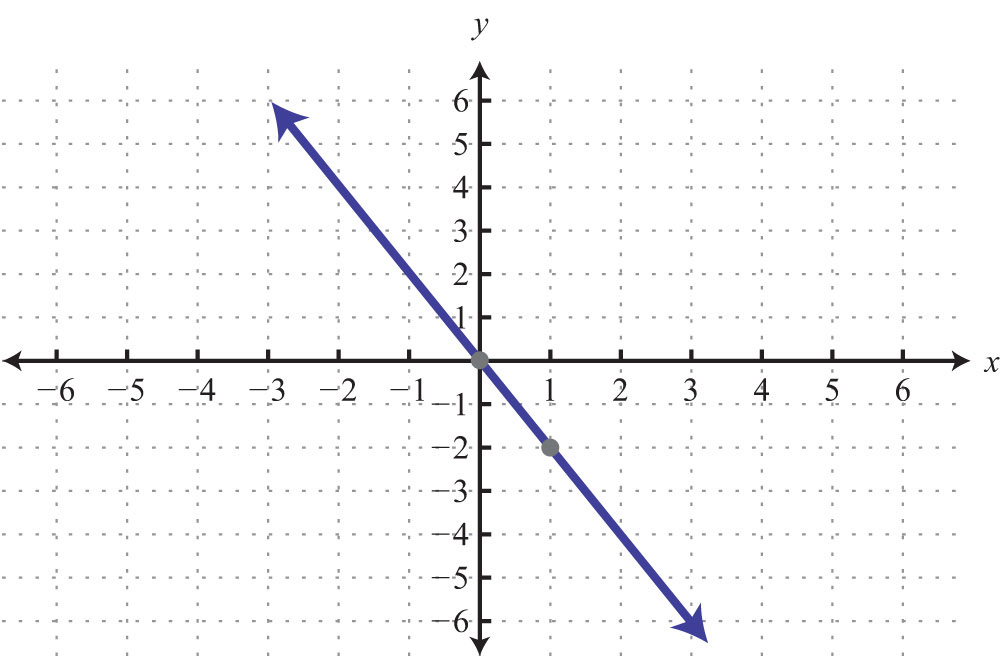53: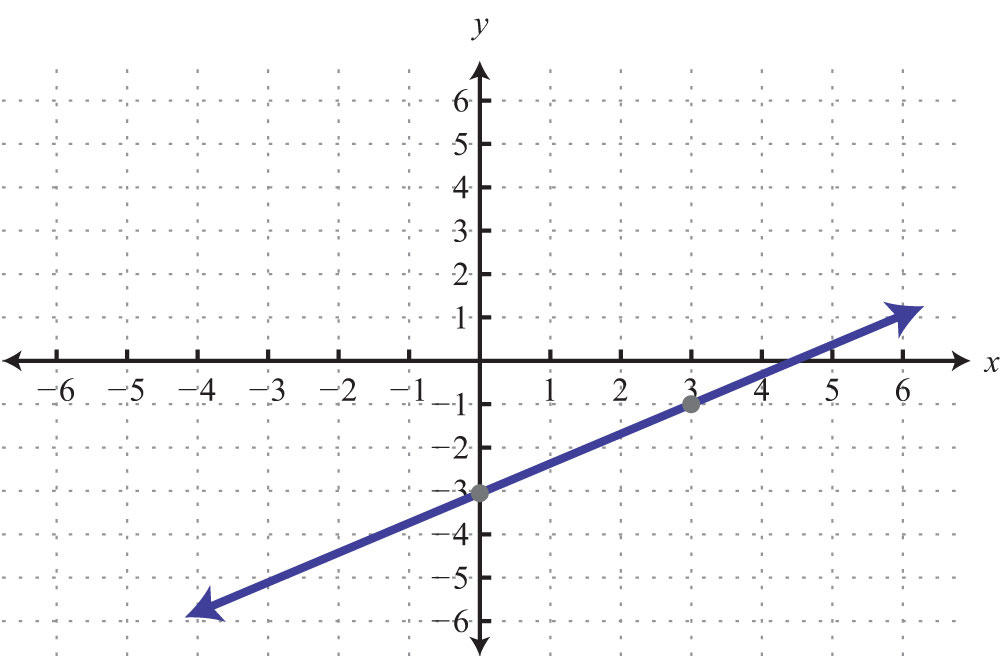55: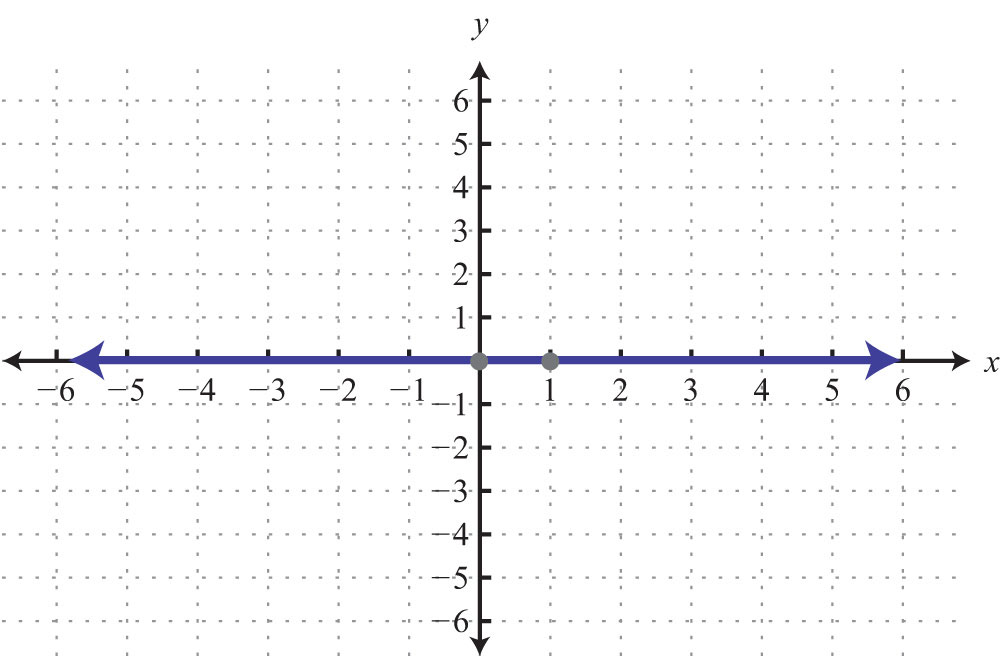57: $y=−2x+1$

59: $y=−5$

61: $y=12x+10$

63: $y=23x−83$

65: $y=45x−1$

67: $y=−34x+12$

69: $y=−6$

71: Parallel

73: Perpendicular

75: $y=5x+49$

77: $y=−34x+5$

79: $y=−1$

81: Domain: {−10, −5, 5}; range: {−1, 2}; function: yes

83: Domain: R; range: R; function: yes

85: Domain: $[−3,∞)$; range: R; function: no

87: $f(−1)=−13$

89: $g(−13)=−12$

91: $x=49$

93: $x=83$

95: $f(3)=−2$

97: Yes

99: No

101: No

103: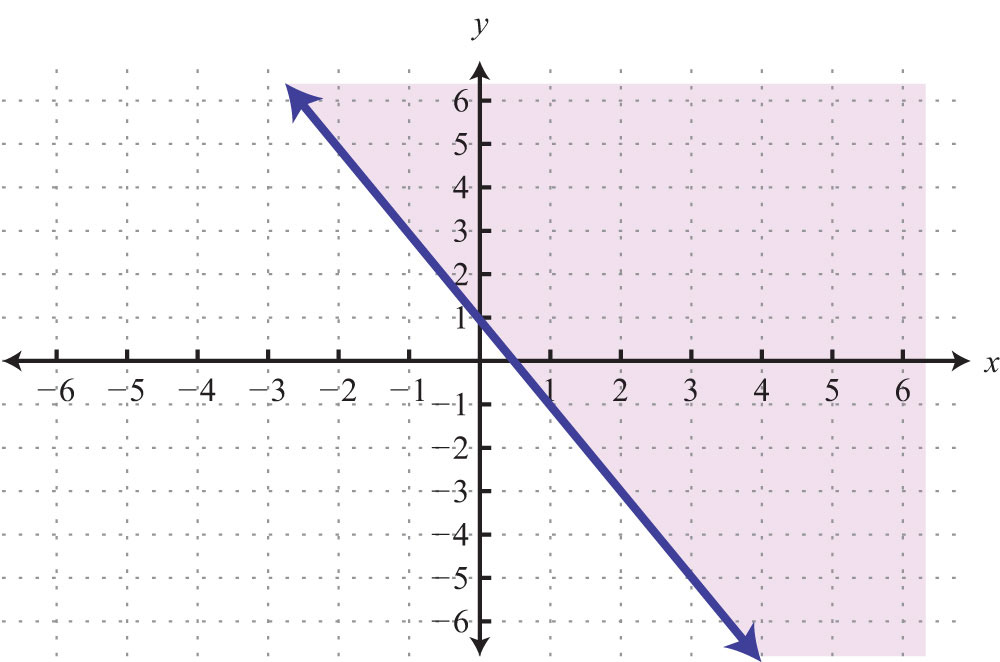105: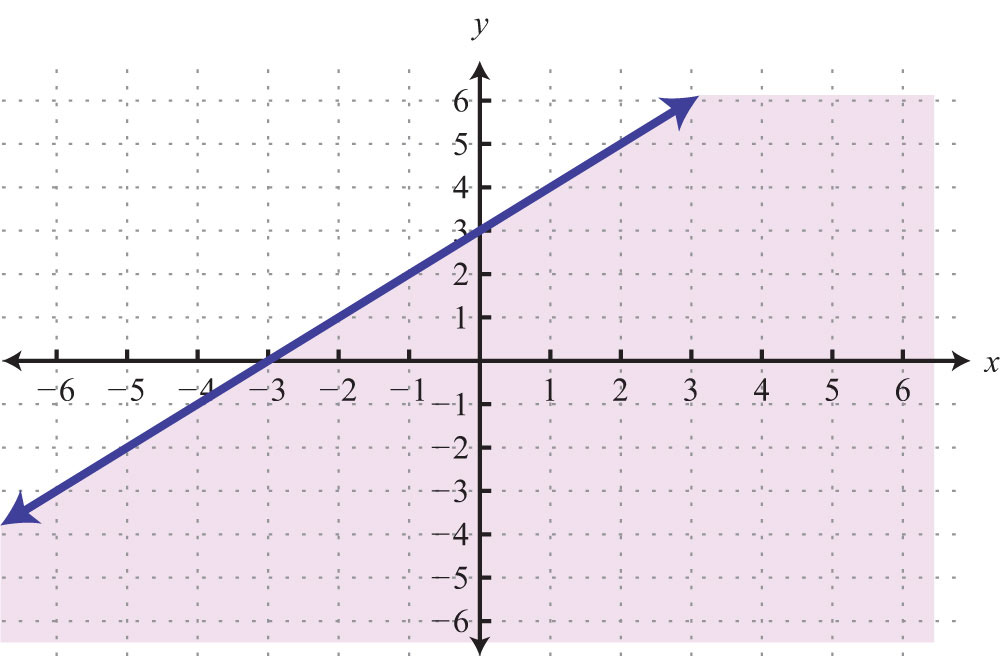107: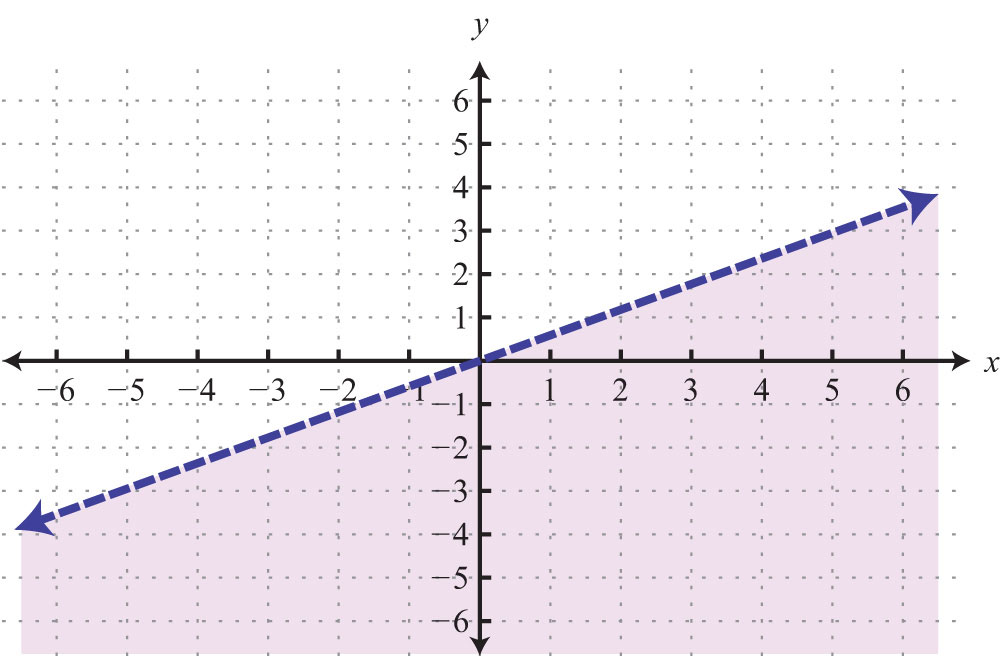1: Area: 6 square units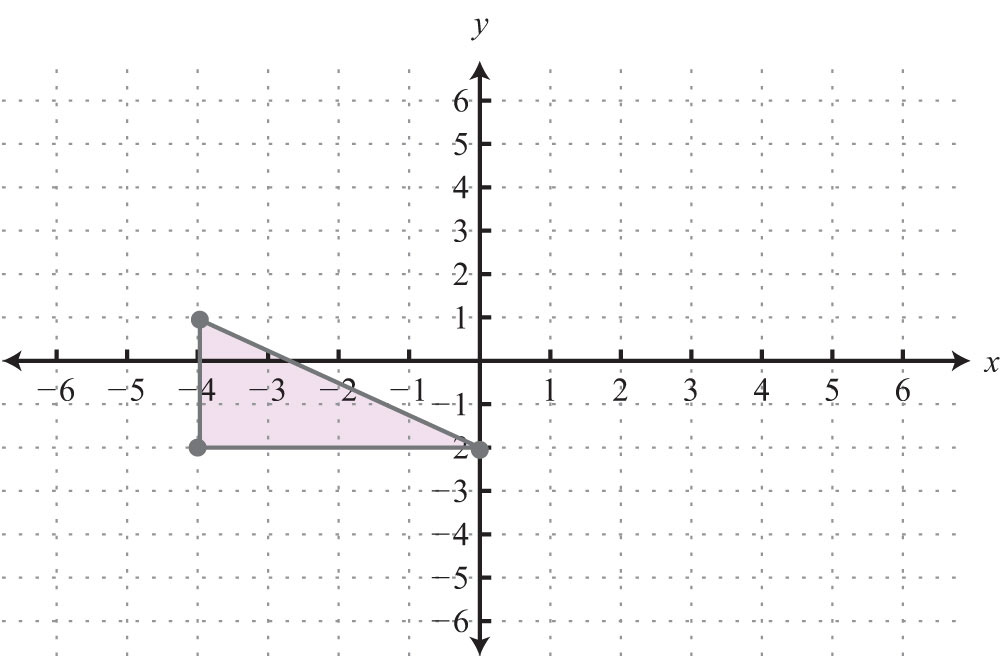3: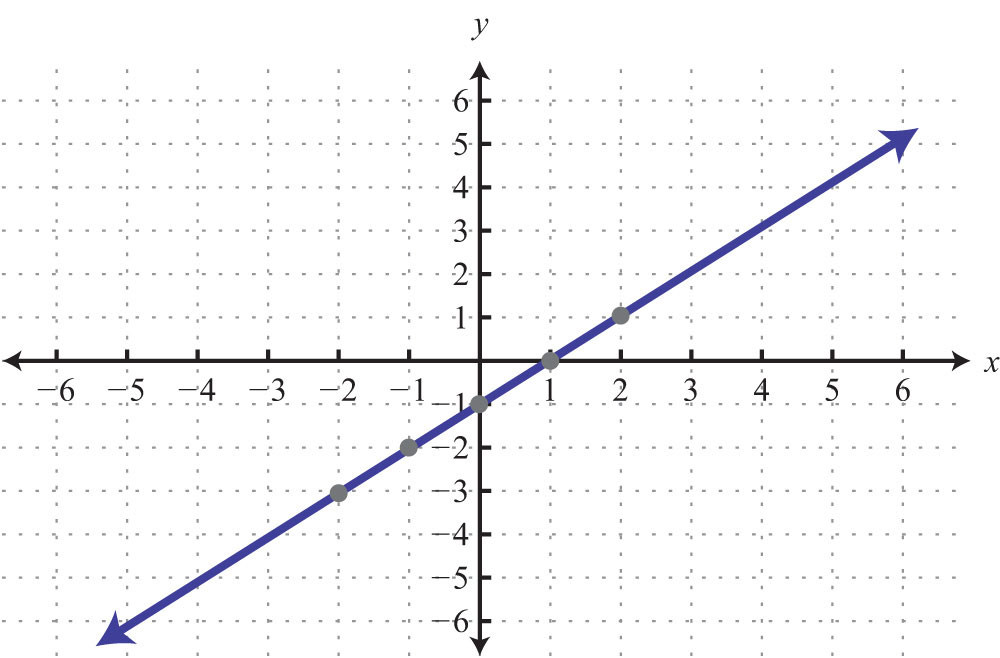5: Intersection: (−3, 4)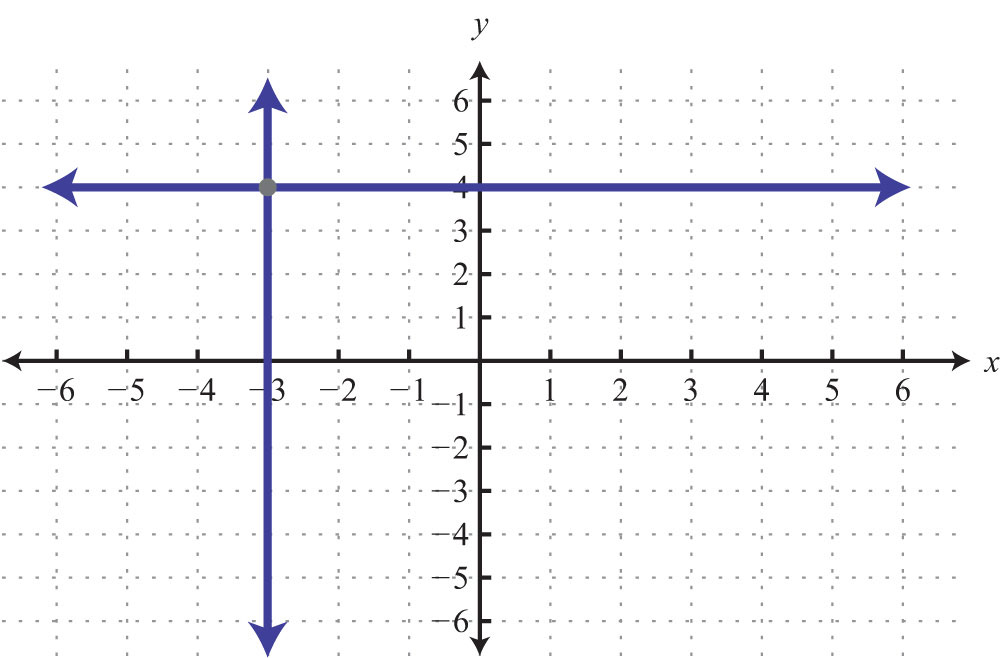7: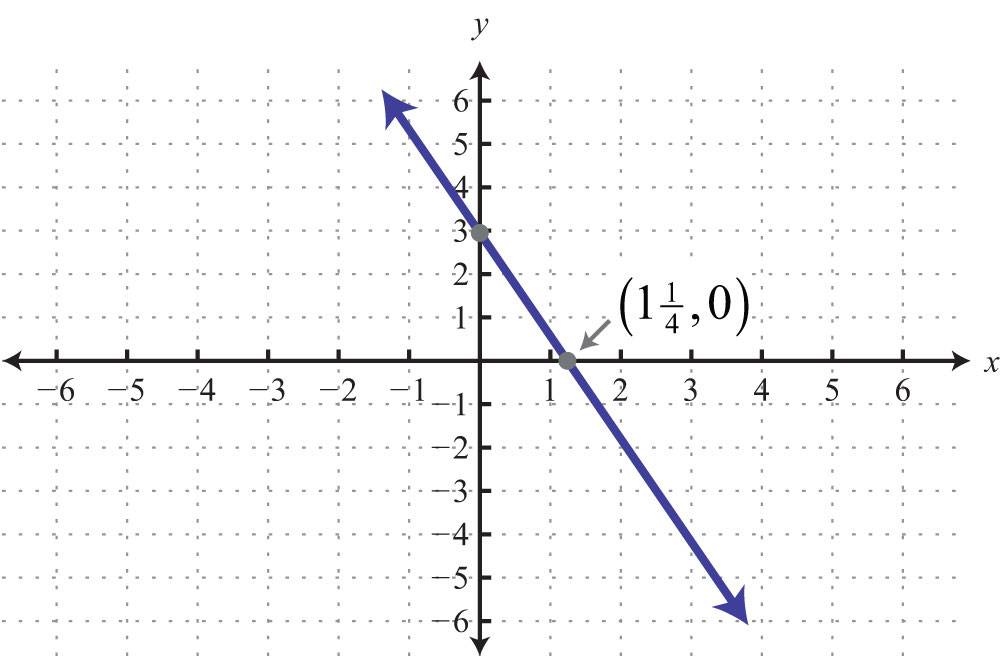9: Slope: −3/2; y-intercept: (0, 6)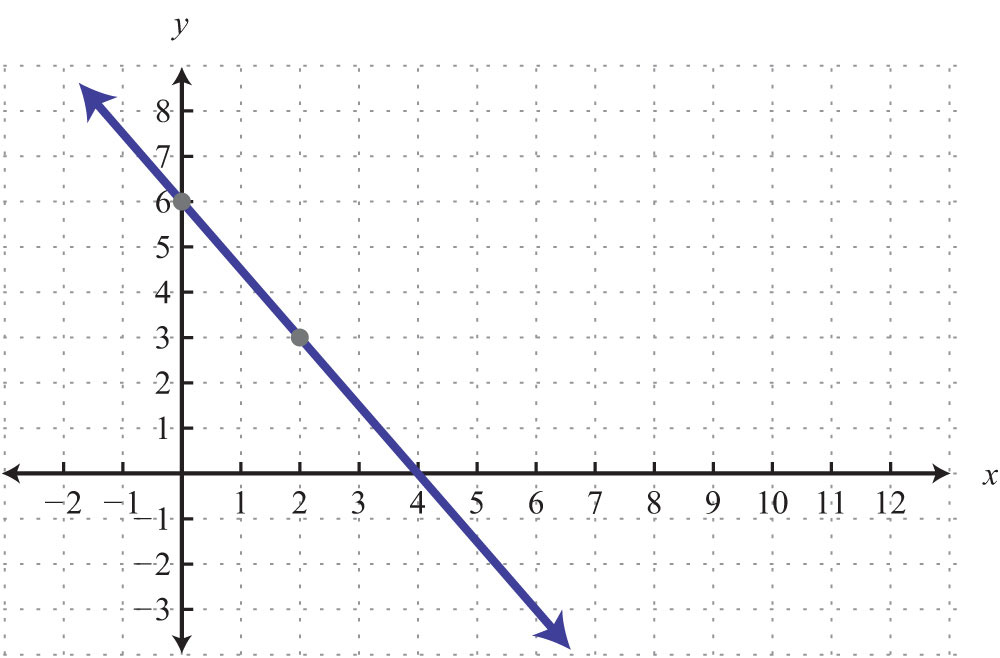11: $m⊥=13$

13:a. 0; b. Undefined; c. Perpendicular

15: $y=−34x+7$

17: $y=5x+3$

19: $f(10)=−6$

21: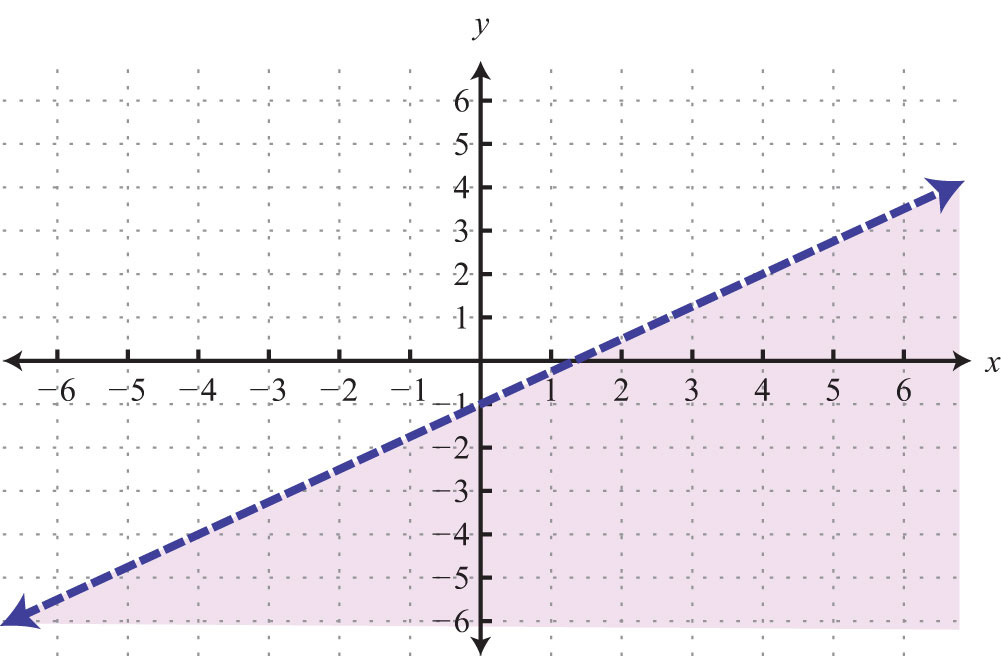23: $cost=0.52x+32$; \$55.92

25: 18 meters